# NCERT Solutions for Class 8 Maths Chapter 15 Exercise 15.3 – Introduction to Graphs

NCERT Solutions for Class 8 Maths Chapter 15 Exercise 15.3 – Introduction to Graphs, has been designed by the NCERT to test the knowledge of the student on the topic – Some Applications of Introduction to Graphs

### NCERT Solutions for Class 8 Maths Chapter 15 Exercise 15.3 – Introduction to Graphs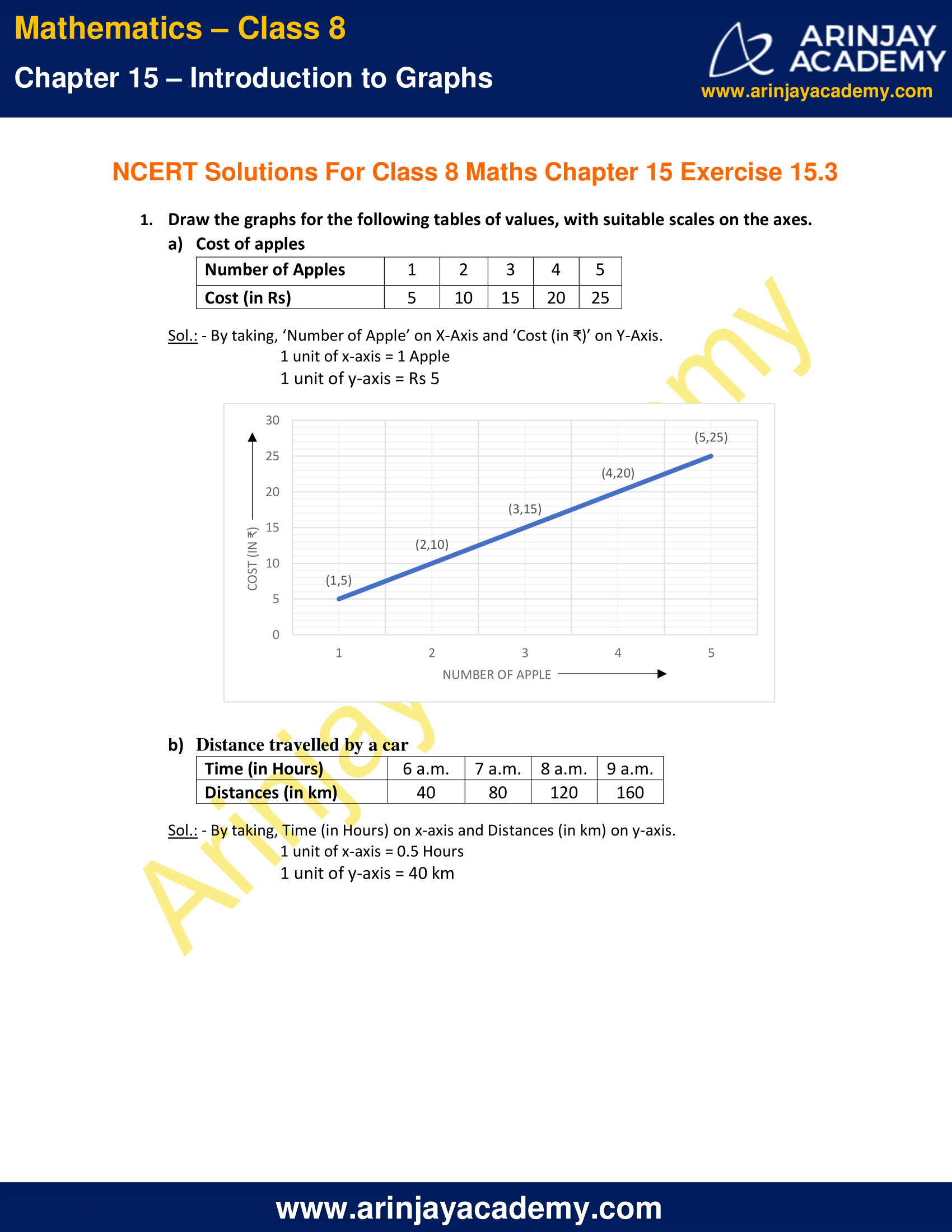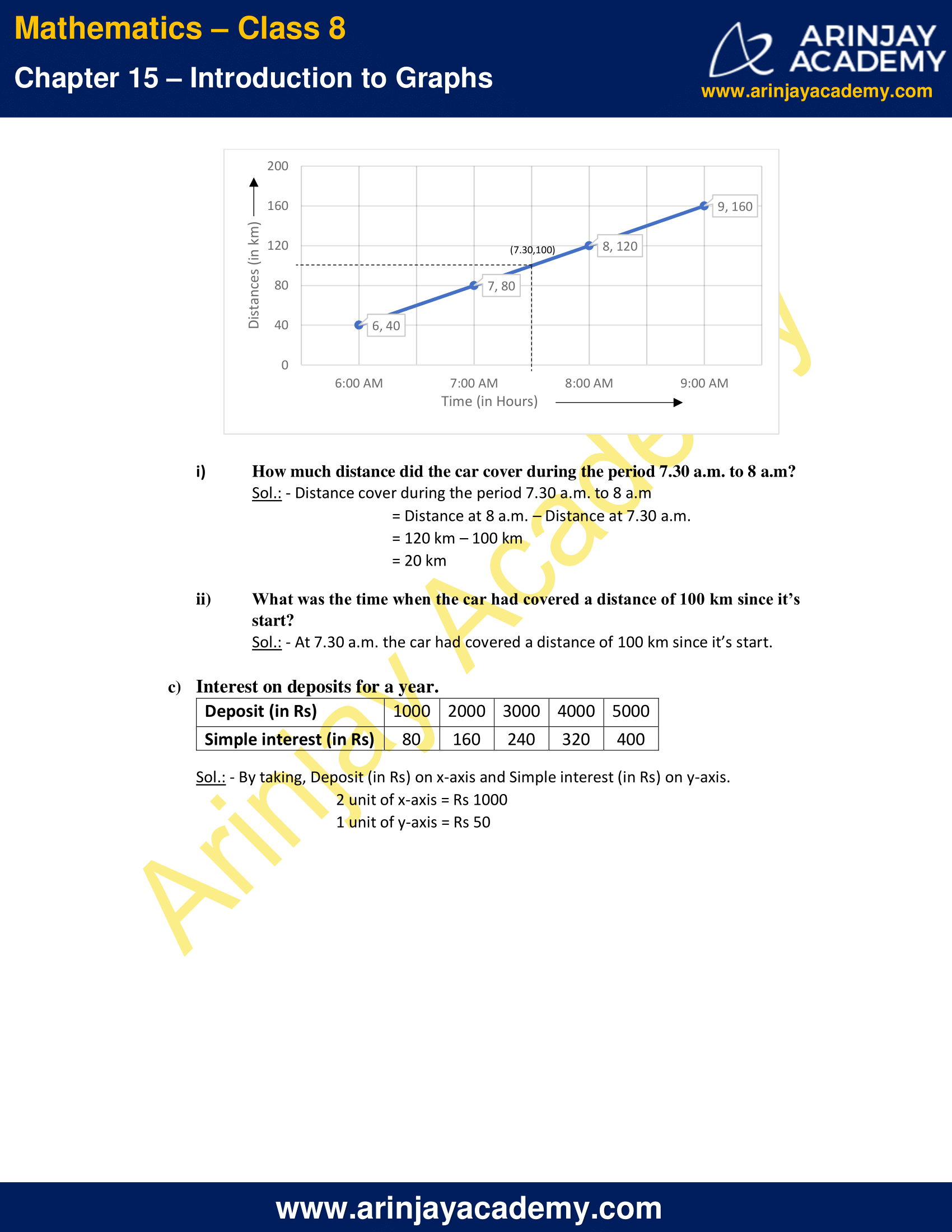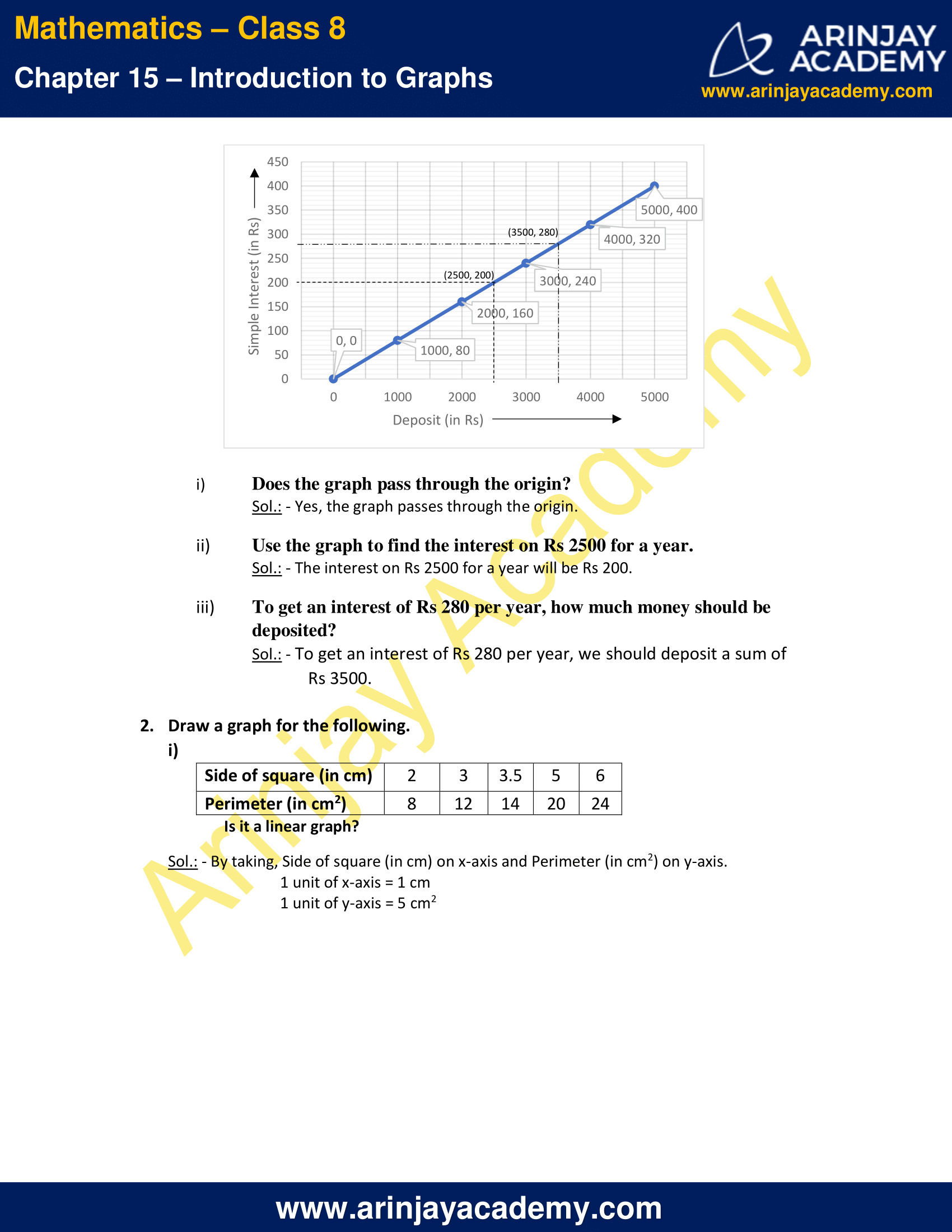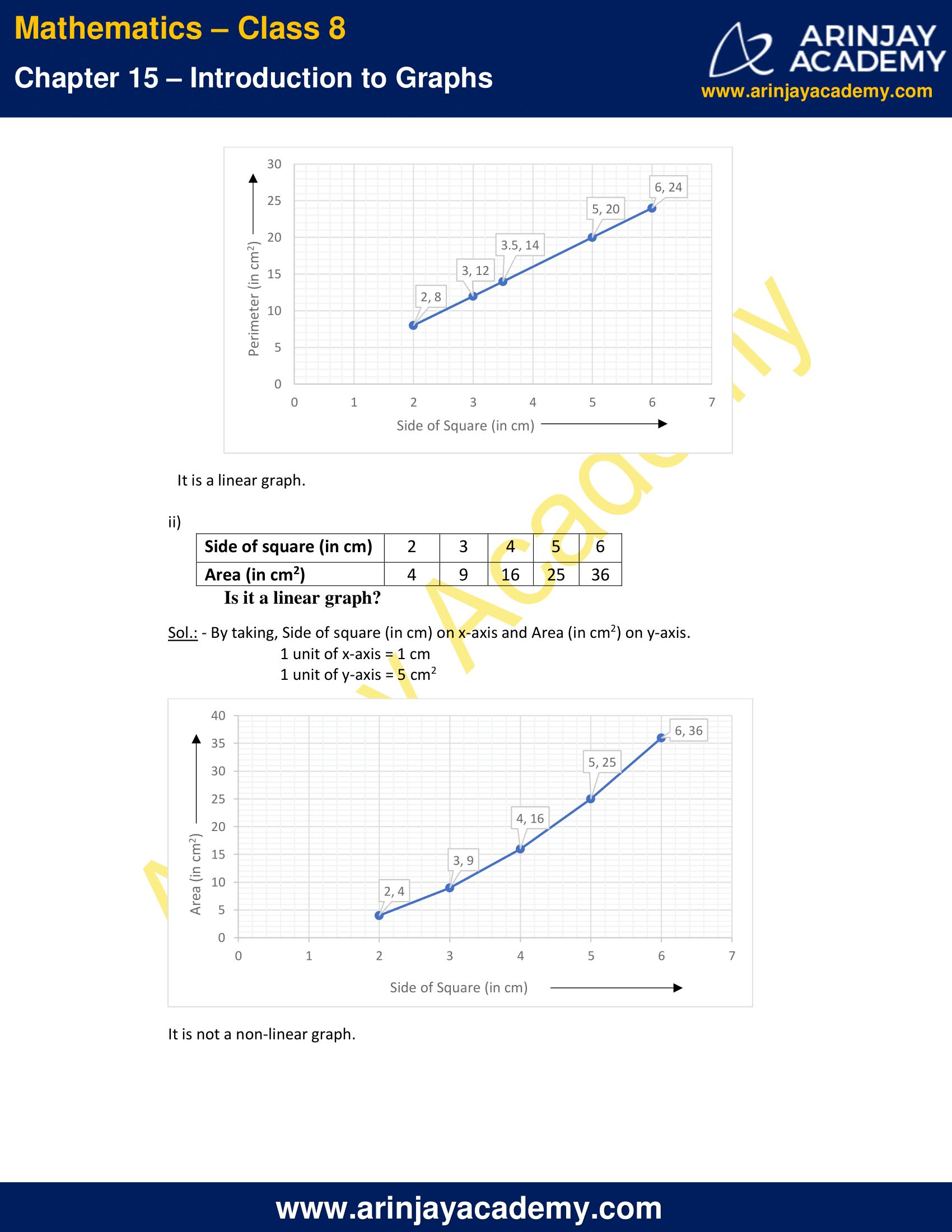NCERT Solutions for Class 8 Maths Chapter 15 Exercise 15.3 – Introduction to Graphs

Draw the graphs for the following tables of values, with suitable scales on the axes.

a) Cost of apples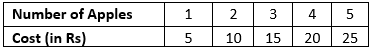Sol.: – By taking, ‘Number of Apple’ on X-Axis and ‘Cost (in ₹)’ on Y-Axis.
1 unit of x-axis = 1 Apple
1 unit of y-axis = Rs 5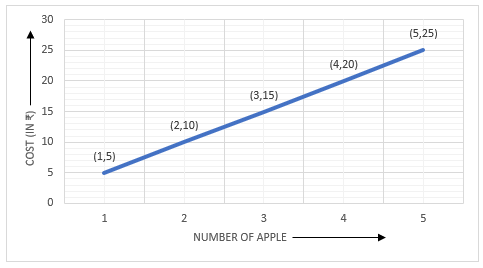b) Distance travelled by a carSol.: – By taking, Time (in Hours) on x-axis and Distances (in km) on y-axis.
1 unit of x-axis = 0.5 Hours
1 unit of y-axis = 40 km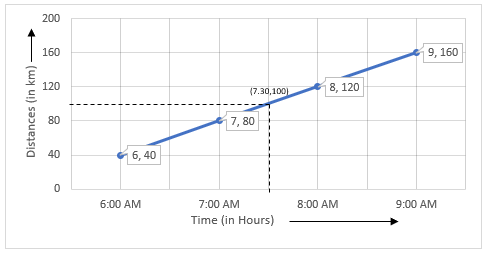i) How much distance did the car cover during the period 7.30 a.m. to 8 a.m?

Sol.: – Distance cover during the period 7.30 a.m. to 8 a.m
= Distance at 8 a.m. – Distance at 7.30 a.m.
= 120 km – 100 km
= 20 km

ii) What was the time when the car had covered a distance of 100 km since it’s start?

Sol.: – At 7.30 a.m. the car had covered a distance of 100 km since it’s start.

c) Interest on deposits for a year.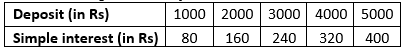Sol.: – By taking, Deposit (in Rs) on x-axis and Simple interest (in Rs) on y-axis.
2 unit of x-axis = Rs 1000
1 unit of y-axis = Rs 50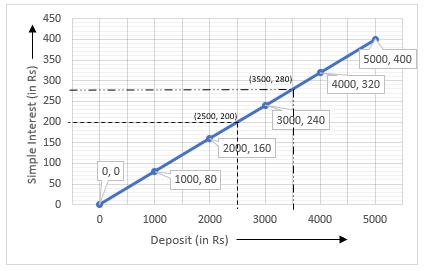i) Does the graph pass through the origin?
Sol.: – Yes, the graph passes through the origin.

ii) Use the graph to find the interest on Rs 2500 for a year.
Sol.: – The interest on Rs 2500 for a year will be Rs 200.

iii) To get an interest of Rs 280 per year, how much money should be deposited?
Sol.: – To get an interest of Rs 280 per year, we should deposit a sum of Rs 3500.

2. Draw a graph for the following.

i)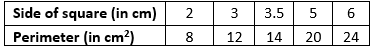Is it a linear graph?

Sol.: – By taking, Side of square (in cm) on x-axis and Perimeter (in cm2) on y-axis.
1 unit of x-axis = 1 cm
1 unit of y-axis = 5 cm2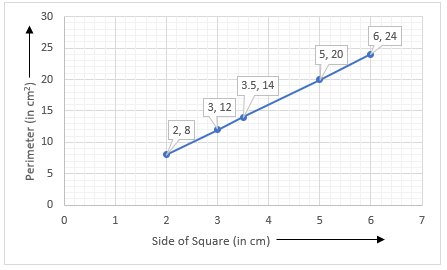It is a linear graph.

ii)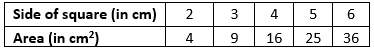Is it a linear graph?

Sol.: – By taking, Side of square (in cm) on x-axis and Area (in cm2) on y-axis.
1 unit of x-axis = 1 cm
1 unit of y-axis = 5 cm2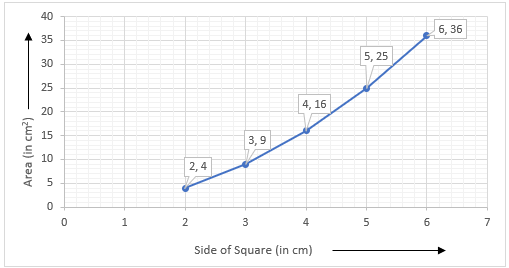It is not a non-linear graph.

The next Exercise for NCERT Solutions for Class 8 Maths Chapter 16 Exercise 16.1 – Playing with Numbers can be accessed by clicking here

Download NCERT Solutions for Class 8 Maths Chapter 15 Exercise 15.3 – Introduction to Graphs